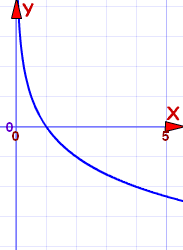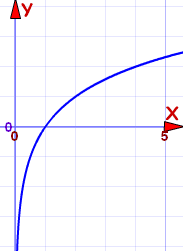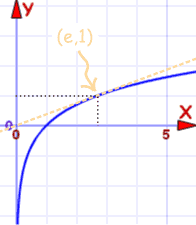# 对数函数参考

f(x) = loga(x)

a 是任何大于 0 的数，但不能等于 1

### 属性视乎 "a" 的值而定

• 如果 a=1，函数是未定义的
• 此外，有两个情形需要考虑：

### a 大于 1a 在 0 与 1 之间

• x 越趋近 0，函数就越趋向无穷大
• x 的值越大，函数就越趋向−无穷大
• 它是个严格递减函数
• y轴（x=0）是函数的垂直渐近线

For a 大于 1：

• x 趋近 0，函数就越趋向−无穷大
• x 的值越大，函数就越趋向无穷大
• 它是个严格递增函数
• y轴（x=0）是函数的垂直渐近线

### 一般来说：

• 对数函数的曲线永远在 y轴右边的一半（x 大于 0），永不穿过 y轴
• 函数的曲线与 x轴在 x=1 相交，就是说，穿过 (1,0)
• x=af(x)=1 …… 就是说，曲线穿过 (a,1)
• 单射（一对一）函数

## 反函数

loga(x) 是 ax指数函数）的反函数

## 自然对数函数

f(x) = loge(x)

f(x) = ln(x)

"ln" 的意思是 "对数，自然"f(x) = ln(x) 的图

(e,1)，曲线的坡度是 1/e，并且线与曲线相切。